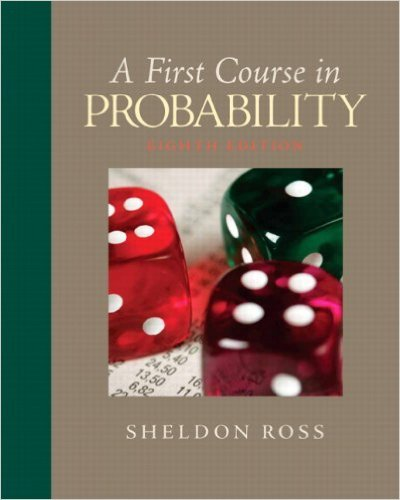×
×

# A, B, and C take turns flipping a coin. The first onetoISBN: 9780136033134 163

## Solution for problem 4 Chapter 2

First Course in Probability | 8th Edition

• Textbook Solutions
• 2901 Step-by-step solutions solved by professors and subject experts
• Get 24/7 help from StudySoup virtual teaching assistantsFirst Course in Probability | 8th Edition

4 5 0 244 Reviews
24
4
Problem 4

A, B, and C take turns flipping a coin. The first oneto get a head wins. The sample space of this experimentcan be defined byS =%1, 01, 001, 0001, . . . ,0000 (a) Interpret the sample space.(b) Define the following events in terms of S:(i) A wins = A.(ii) B wins = B.(iii) (A B)c.Assume that A flips first, then B, then C,then A, and so on.

Step-by-Step Solution:
Step 1 of 3

X, Y Bivariate Random Variables f(u1, 2 ) is density of (X,Y) ∫ ( ) density of X ∫ ( ) density of Y X, Y are independent if f(u1, 2 = f1(u1 2 (u2) If X1, ,2…….X are nndependent Var(X 1 ……..X ) = n + …….. We want to produce to specification “m” “m” = length of a part, content of juice can etc We want to check at the...

Step 2 of 3

Step 3 of 3

##### ISBN: 9780136033134

Unlock Textbook Solution